#### A PHP Error was encountered

Severity: Notice

Message: Trying to get property of non-object

Line Number: 17

Backtrace:

Line: 17
Function: _error_handler

File: /home/publishers12/public_html/application/controllers/Articleviews.php
Line: 60
Function: view

File: /home/publishers12/public_html/index.php
Line: 318
Function: require_once

#### A PHP Error was encountered

Severity: Notice

Message: Trying to get property of non-object

Line Number: 18

Backtrace:

Line: 18
Function: _error_handler

File: /home/publishers12/public_html/application/controllers/Articleviews.php
Line: 60
Function: view

File: /home/publishers12/public_html/index.php
Line: 318
Function: require_once

#### A PHP Error was encountered

Severity: Notice

Message: Trying to get property of non-object

Line Number: 19

Backtrace:

Line: 19
Function: _error_handler

File: /home/publishers12/public_html/application/controllers/Articleviews.php
Line: 60
Function: view

File: /home/publishers12/public_html/index.php
Line: 318
Function: require_once

#### A PHP Error was encountered

Severity: Notice

Message: Trying to get property of non-object

Line Number: 20

Backtrace:

Line: 20
Function: _error_handler

File: /home/publishers12/public_html/application/controllers/Articleviews.php
Line: 60
Function: view

File: /home/publishers12/public_html/index.php
Line: 318
Function: require_once

Electro kinetic fractal dimension for characterizing shajara reservoirs of the permocarboniferous shajara formation saudi arabia

#### A PHP Error was encountered

Severity: Notice

Message: Trying to get property of non-object

Line Number: 35

Backtrace:

Line: 35
Function: _error_handler

File: /home/publishers12/public_html/application/controllers/Articleviews.php
Line: 60
Function: view

File: /home/publishers12/public_html/index.php
Line: 318
Function: require_once

">

#### A PHP Error was encountered

Severity: Notice

Message: Trying to get property of non-object

Line Number: 36

Backtrace:

Line: 36
Function: _error_handler

File: /home/publishers12/public_html/application/controllers/Articleviews.php
Line: 60
Function: view

File: /home/publishers12/public_html/index.php
Line: 318
Function: require_once

">

## "Electro Kinetic Fractal Dimension for Characterizing Shajara Reservoirs of the Permo-Carboniferous Shajara Formation, Saudi Arabia"

https://www.gavinpublishers.com/journals/journals_details/" target="_blank">  Home
• #### A PHP Error was encountered

Severity: Notice

Message: Trying to get property of non-object

Filename: views/article_view.php

Line Number: 39

Backtrace:

File: /home/publishers12/public_html/application/views/article_view.php
Line: 39
Function: _error_handler

File: /home/publishers12/public_html/application/controllers/Articleviews.php
Line: 62
Function: view

File: /home/publishers12/public_html/index.php
Line: 318
Function: require_once

• " target="_blank">   Board Members
• #### A PHP Error was encountered

Severity: Notice

Message: Trying to get property of non-object

Filename: views/article_view.php

Line Number: 40

Backtrace:

File: /home/publishers12/public_html/application/views/article_view.php
Line: 40
Function: _error_handler

File: /home/publishers12/public_html/application/controllers/Articleviews.php
Line: 62
Function: view

File: /home/publishers12/public_html/index.php
Line: 318
Function: require_once

• " target="_blank">   Article In Press
• #### A PHP Error was encountered

Severity: Notice

Message: Trying to get property of non-object

Filename: views/article_view.php

Line Number: 41

Backtrace:

File: /home/publishers12/public_html/application/views/article_view.php
Line: 41
Function: _error_handler

File: /home/publishers12/public_html/application/controllers/Articleviews.php
Line: 62
Function: view

File: /home/publishers12/public_html/index.php
Line: 318
Function: require_once

" target="_blank">   Current Issue
• #### A PHP Error was encountered

Severity: Notice

Message: Trying to get property of non-object

Filename: views/article_view.php

Line Number: 42

Backtrace:

File: /home/publishers12/public_html/application/views/article_view.php
Line: 42
Function: _error_handler

File: /home/publishers12/public_html/application/controllers/Articleviews.php
Line: 62
Function: view

File: /home/publishers12/public_html/index.php
Line: 318
Function: require_once

" target="_blank">   Archive
• #### A PHP Error was encountered

Severity: Notice

Message: Trying to get property of non-object

Filename: views/article_view.php

Line Number: 43

Backtrace:

File: /home/publishers12/public_html/application/views/article_view.php
Line: 43
Function: _error_handler

File: /home/publishers12/public_html/application/controllers/Articleviews.php
Line: 62
Function: view

File: /home/publishers12/public_html/index.php
Line: 318
Function: require_once

" target="_blank">  Article Process Fee
• #### A PHP Error was encountered

Severity: Notice

Message: Trying to get property of non-object

Filename: views/article_view.php

Line Number: 44

Backtrace:

File: /home/publishers12/public_html/application/views/article_view.php
Line: 44
Function: _error_handler

File: /home/publishers12/public_html/application/controllers/Articleviews.php
Line: 62
Function: view

File: /home/publishers12/public_html/index.php
Line: 318
Function: require_once

" target="_blank">   Special Issue
• Khalid Elyas Mohamed Elameen Alkhidir*

Department of Petroleum and Natural Gas Engineering, King Saud University, Riyadh, Saudi Arabia

*Corresponding author: Khalid Elyas Mohamed Elameen Alkhidir, Department of Petroleum and Natural Gas Engineering, College of Engineering, King Saud University, Saudi Arabia. Tel: +966114679118; Email: kalkhidir@ksu.edu.sa

Received Date: 25 April, 2018; Accepted Date: 02 May, 2018; Published Date: 10 May, 2018

1.       Abstract

Electro kinetic parameters such as streaming potential coupling coefficient, electro osmosis coupling coefficient, and their ratio play an important role in the determination of quality and assessment of a reservoir. This research aims to calculate fractal dimension from the relationship among the ratio of streaming potential to electro osmosis coupling coefficient, maximum streaming potential to electro osmosis coupling coefficient ratio and water saturation and to confirm it by the fractal dimension derived from the relationship among capillary pressure and water saturation. The ratio of streaming potential to electro osmosis coupling coefficient was determined from effective pore radius, fluid viscosity, and fluid electric conductivity. The effective pore radius of the real collected sandstone samples was calculated from packing constant and pore radius determined from capillary pressure measured by mercury intrusion technique which enables measurement of porosity and calculation of permeability. Two equations for calculating the fractal dimensions have been employed. The first one describes the functional relationship between water saturation, ratio of streaming potential to electro osmosis coupling coefficient, maximum streaming potential to electro osmosis coefficient ratio and fractal dimension. The second equation implies to the water saturation as a function of capillary pressure and the fractal dimension. Two procedures for obtaining the fractal dimension have been utilized. The first procedure was done by plotting the logarithm of the ratio between streaming potential to electro osmosis coupling coefficient divided by the logarithm of maximum value describing the ratio between streaming potential and electro osmosis coupling coefficient versus logarithm water saturation. The slope of the first procedure is positive = 3- Df (fractal dimension). The second procedure for obtaining the fractal dimension was resolved by plotting the logarithm of capillary pressure versus the logarithm of water saturation. The slope of the second procedure is negative = Df -3. On the basis of the obtained results of the constructed stratigraphic column and the attained values of the fractal dimension, the sandstones of the Shajara reservoirs of the Shajara Formation were divided here into three units. It was also noted that samples with wide range of pore radius were characterized by high values of fractal dimensions due to an increase in their connectivities and effective pore radius.

2.       Keywords: Electro Kinetic Fractal Dimension; Shajara Formation; Shajara Reservoirs

1.       Introduction

An increase of electro kinetic coupling coefficient with increasing permeability that can explain by the effect of variation in surface conductivity was examined by . The reduction of streaming potential coefficient during compaction suggests that the tortuosity of the hydraulic network increases faster than tortuosity of the electric network was reported by . An increase of streaming potential coupling coefficient with increasing permeability was demonstrated by . A new method to calculate total electric conductivity from the ratio of pore area cross-section to length of the effective rock capillaries which derived from streaming potential measurements was investigated by . Toledo et al 1994 in Li and Williams 2007  reported that capillary pressure follows the scaling law at low water saturation. Streaming potential coupling coefficient measurements to monitor flow in saline surface environments such as deep saline aquifers and hydrocarbon reservoirs was studied by . Electro kinetic relationship to produce a function that links the mean grain size of a rock to its effective mean pore radius was described by . An increase of volumetric flow rate, which, in turn, increases the penetration distance of the acid before it is being spent due to application of direct current to improve acidizing operations was investigated by . A novel method for estimating permeability with streaming current and electro osmosis pressure was developed by . Good agreement between electro kinetically deduced and gas measured permeability was presented by . An increase of bubble pressure fractal dimension and pressure head fractal dimension with decreasing pore size distribution index and fitting parameters m×n due to possibility of having inter connected channels was proofed by . An increase of fractal dimension with increasing permeability, relaxation time of induced polarization, due to an increase in pore connectivity was confirmed by .

2.       Experimental Procedure

Two procedures for obtaining the fractal dimension have been utilized. The first procedure was completed by plotting the logarithm of the ratio between streaming potential to electro osmosis coupling coefficient divided by the logarithm of maximum value describing the ratio between streaming potential and electro osmosis coupling coefficient versus logarithm water saturation. The slope of the first procedure is positive = 3- Df (fractal dimension). The second procedure for obtaining the fractal dimension was determined by plotting the logarithm of capillary pressure versus the logarithm of water saturation. The slope of the second procedure is negative = Df -3.

3.       Materials and Methods

Samples were collected from the Surface type section of the Shajara Reservoirs of Permo-Carboniferous Shajara Formation, latitude 26° 52' 17.4,"longitude 43° 36' 18" (Figure 1). Porosity was measured and permeably was calculated from the measured capillary pressure data obtained by mercury intrusion technique on powder sandstone samples.  Effective pore radius was determined from distribution of pores which enables the calculation of electro kinetic fractal dimension.

The electro kinetic fractal dimension can be scaled as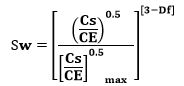1

Sw= water saturation.

Cs= streaming potential coefficient in volt / pascal

CE =electro osmosis coefficient in pascal / volt

Df the fractal dimension.

Equation 1 can be proofed from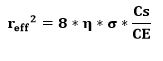2

reff = effective pore radius in meter

η=fluid viscosity in pascal. Second

σ=fluid electric conductivity in Siemens per meter.

Take the square root of equation 2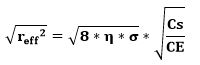3

Equation 3 after simplification will become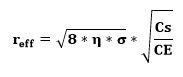4

The effective pore radius can be scaled as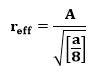5

a=packing constant

Insert equation 5 into equation 4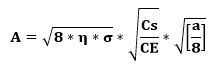6

The maximum pore radius can be scaled as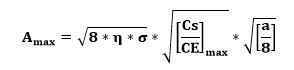7

Divide equation 6 by equation 7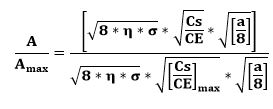8

Equation 8 after simplification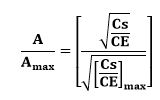9

Take the logarithm of equation 9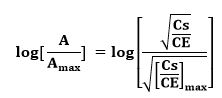10

Insert equation 11 into equation 10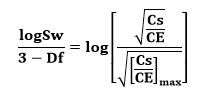12

Remove the logarithm from equation 12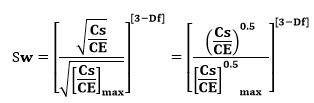13

Equation 13 the proof of equation 1 which relates the water saturation, the ratio of streaming potential coefficient to electro osmosis coefficient and the fractal dimension.

The capillary pressure fractal dimension was determined from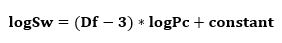14

Where Sw the water saturation Pc the capillary pressure and Df the fractal dimension

4.       Results and Discussion

Based on field observation the Shajara Reservoirs of the Permo-Carboniferous Shajara Formation were divided here into three units as explained in (Figure 1). These units from bottom to top are: Lower, Middle and Upper Shajara Reservoir. Their acquired results of electro kinetic and capillary fractal dimensions are presented in (Table 1). The results show equalities between electro kinetic fractal dimension and capillary pressure fractal dimension. A maximum fractal dimension value of about 2.7872 was reported from sample SJ13 as illustrated in (Table 1). However, a minimum fractal dimension value 2.4379 allocates to sample SJ3 from the Lower Shajara Reservoir as delineated in (Table 1). The electro kinetic and capillary pressure fractal dimensions were noticed to increase with increasing permeability due to the possibility of having interconnected channels as verified in (Table 1).

Regarding the Lower Shajara Reservoir, it is represented by six sandstone samples as shown in (Figure 1), four of which label as SJ1, SJ2, SJ3, and SJ4 were selected for capillary pressure measurements to determine the fractal dimension. Their positive slopes of the first procedure (log ratio of streaming potential to electro osmosis coupling coefficient divided by maximum streaming potential to electro osmosis coefficient ratio versus log water saturation (Sw) and negative slopes of the second procedure (log capillary pressure versus log water saturation are delineated in (Figure 2), (Figure 3), (Figure 4), (Figure 5) and (Table 1). Their electro kinetic fractal dimension and capillary pressure fractal dimension values are shown in Table 1. As we progress from sample SJ2 to SJ3 a remarkable reduction in permeability from 1955 md to 56 md was observed due to compaction which reflects change in electro kinetic fractal dimension from 2.7748 to 2.4379 as demonstrated in (Table 1). Such drastic change in permeability can account for heterogeneity which is a key parameter in reservoir quality assessment. Again, an increase in grain size and permeability was reported from sample SJ4 whose electro kinetic fractal dimension and capillary pressure fractal dimension was found to be 2.6843 as demonstrated in (Table 1).

Concerning the Middle Shajara Reservoir, it is separated from Lower Shajara Reservoir by an unconformity surface as shown in (Figure 1). It was represented by four samples, three of which named as SJ7, SJ8, and SJ9 were chosen for fractal dimension determination as specified in (Table 1). Their positive and negative slopes of the first and second procedures were presented in (Figure 6), (Figure 7), and (Figure 8) and (Table 1) Additionally, their electro kinetic and capillary pressure fractal dimension exhibit equal values as displayed in (Table 1) Moreover their fractal dimension values are higher than those of sample SJ3 and SJ4 from the Lower Shajara Reservoir due to an increase in their permeability as illustrated in (Table 1).

On the other hand, the Upper Shajara reservoir is separated from the Middle Shajara reservoir by yellow green mudstone as revealed in (Figure 1). It is defined by three sam­ples so called SJ11, SJ12, SJ13 as explained in (Table 1). Their positive slopes of the first procedure and negative slopes of the second procedure are displayed in (Figure 9), (Figure 10) and (Figure 11) and (Table 1). Moreover, their electro kinetic fractal dimension and capillary pressure fractal dimension are also higher than those of sample SJ3 and SJ4 from the Lower Shajara Reservoir due to an increase in their permeability as clarified in (Table 1).

Overall a plot of positive slope of the first procedure versus negative slope of the second procedure delineates three reservoir zones of varying petrophysical characteristics as shown in (Figure 12). These zones were also confirmed by plotting electro kinetic fractal dimension versus capillary pressure fractal dimension as shown in (Figure 13). Such variation in fractal dimensions can be used to explain heterogeneity which is a key parameter in reservoir quality assessment.

5.       Conclusions

1.       The sandstones of the Shajara Reservoirs of the Permo-Carboniferous Shajara Formation were divided here into three units based on electro kinetic fractal.

2.       The Units from bottom to top are Lower Shajara electro kinetic fractal dimension unit, Middle Shajara electro kinetic fractal dimension unit, and Upper Shajara electro kinetic fractal dimension unit.

3.       These units were also confirmed by capillary pressure fractal dimension

4.       Variation in fractal dimensions can account for heterogeneity which is a key parameter in reservoir quality assessment.

5.       The heterogeneity increases with increasing permeability, increasing fractal dimension, decreasing compaction owing to possibility of having interconnected channels.

6.       Acknowledgements

The author would like to thank college of Engineering, King Saud University, Department of Petroleum and Natural Gas Engineering, Department of Chemical Engineering, Research Centre at college of Engineering and King Abdulla Institute for Research and Consulting Studies for their supports.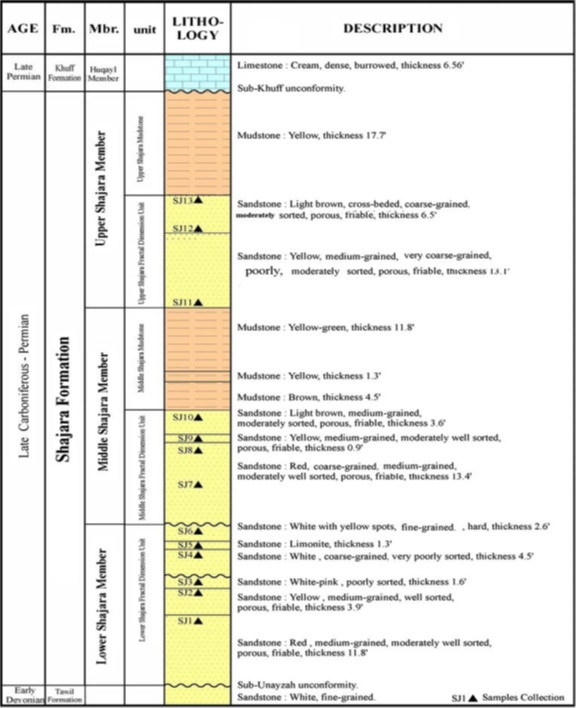Figure 1: Surface type section of the Shajara Reservoirs of Permo-Carboniferous Shajara Formation. Latitude 26° 52' 17.4,"longitude 43° 36' 18".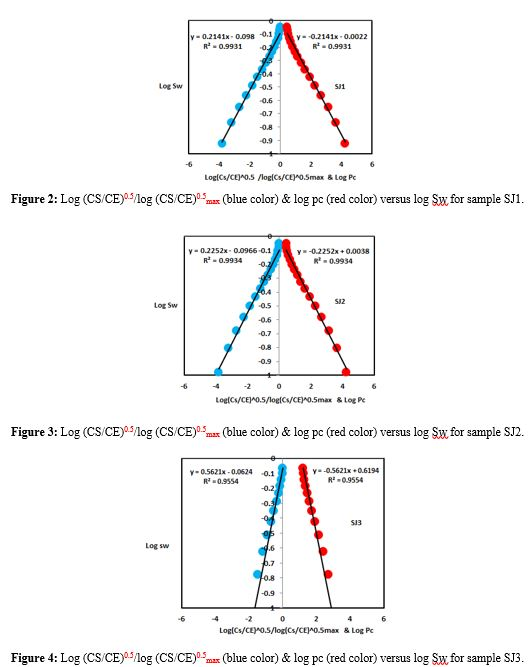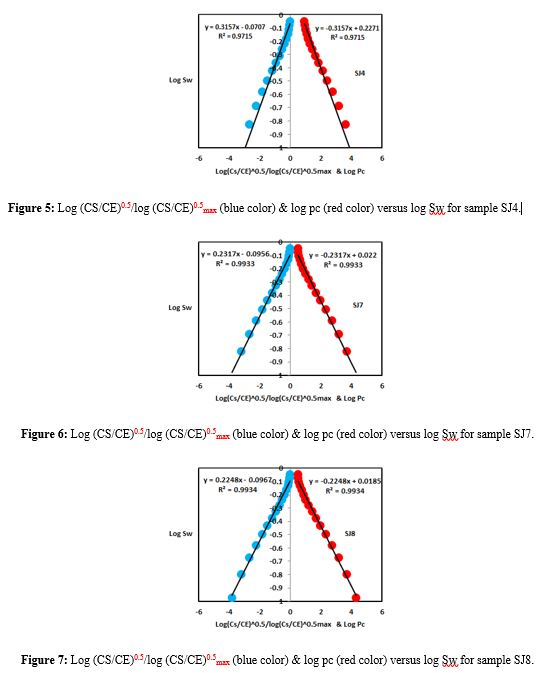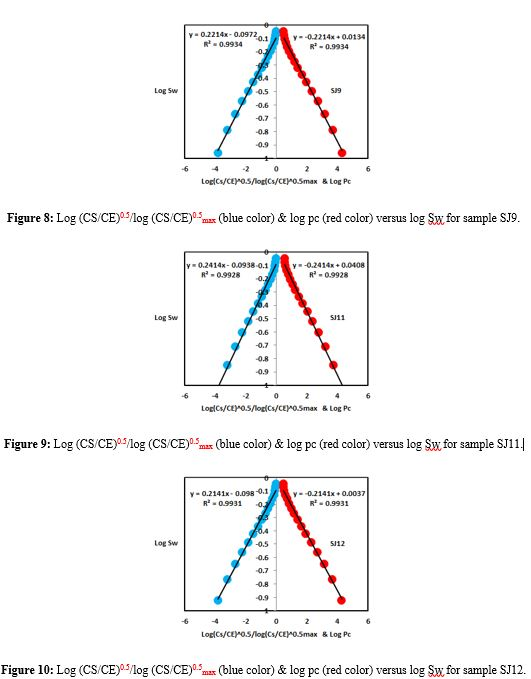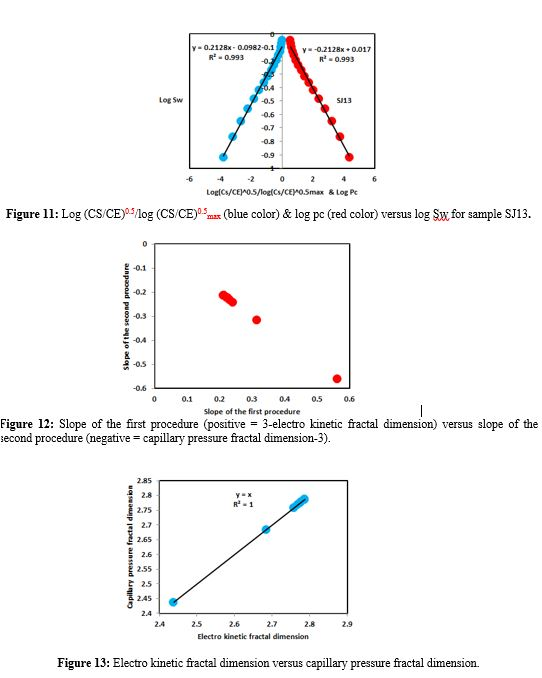Reservoir Sample Porosity % Permeability md Positive Slope of first procedure Slope=3-Df Negative Slope of second procedure Slope =Df-3 Electro kinetic fractal dimension Capillary pressure fractal dimension Upper Shajara Reservoir SJ13 25 973 0.2128 -0.2128 2.7872 2.7872 SJ12 28 1440 0.2141 -0.2141 2.7859 2.7859 SJ11 36 1197 0.2414 -0.2414 2.7586 2.7586 Middle Shajara Reservoir SJ9 31 1394 0.2214 -0.2214 2.7786 2.7786 SJ8 32 1344 0.2248 -0.2248 2.7752 2.7752 SJ7 35 1472 0.2317 -0.2317 2.7683 2.7683 Lower Shajara Reservoir SJ4 30 176 0.3157 -0.3157 2.6843 2.6843 SJ3 34 56 0.5621 -0.5621 2.4379 2.4379 SJ2 35 1955 0.2252 -0.2252 2.7748 2.7748 SJ1 29 1680 0.2141 -0.2141 2.7859 2.7859

Table 1: Petro physical model showing the three Shajara Reservoirs with their corresponding values of electro kinetic and capillary pressure fractal dimension.

Citation: Alkhidir KEME (2018) Electro Kinetic Fractal Dimension for Characterizing Shajara Reservoirs of the Permo-Carboniferous Shajara Formation, Saudi Arabia. Arch Oil Gas Res: AOGR-102. DOI: 10.29011/ AOGR-102. 000002